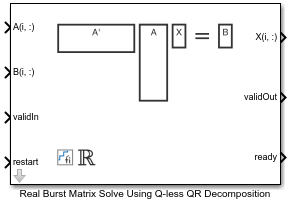# Real Burst Matrix Solve Using Q-less QR Decomposition

Compute the value of X in the equation A'AX = B for real-valued matrices using Q-less QR decomposition

Since R2020a

•Libraries:
Fixed-Point Designer HDL Support / Matrices and Linear Algebra / Linear System Solvers

## Description

The Real Burst Matrix Solve Using Q-less QR Decomposition block solves the system of linear equations A'AX = B using Q-less QR decomposition, where A and B are real-valued matrices.

When Regularization parameter is nonzero, the Real Burst Matrix Solve Using Q-less QR Decomposition block solves the matrix equation

`${\left[\begin{array}{c}\lambda {I}_{n}\\ A\end{array}\right]}^{\text{'}}\cdot \left[\begin{array}{c}\lambda {I}_{n}\\ A\end{array}\right]X=\left({\lambda }^{2}{I}_{n}+A\text{'}A\right)X=B$`

where λ is the regularization parameter, A is an m-by-n matrix, and In = `eye(n)`.

## Ports

### Input

expand all

Rows of real matrix A, specified as a vector. A is an m-by-n matrix where m ≥ 2 and mn. If B is single or double, A must be the same data type as B. If A is a fixed-point data type, A must be signed, use binary-point scaling, and have the same word length as B. Slope-bias representation is not supported for fixed-point data types.

Data Types: `single` | `double` | `fixed point`

Rows of real matrix B, specified as a vector. B is an n-by-p matrix where n ≥ 2. If A is single or double, B must be the same data type as A. If B is a fixed-point data type, B must be signed, use binary-point scaling, and have the same word length as A. Slope-bias representation is not supported for fixed-point data types.

Data Types: `single` | `double` | `fixed point`

Whether inputs are valid, specified as a Boolean scalar. This control signal indicates when the data from the `A(i,:)` and `B(i,:)` input ports are valid. When this value is `1` (`true`) and the value at `ready` is `1` (`true`), the block captures the values at the `A(i,:)` and `B(i,:)` input ports. When this value is `0` (`false`), the block ignores the input samples.

After sending a `true` `validIn` signal, there may be some delay before `ready` is set to `false`. To ensure all data is processed, you must wait until `ready` is set to `false` before sending another `true` `validIn` signal.

Data Types: `Boolean`

Whether to clear internal states, specified as a Boolean scalar. When this value is 1 (`true`), the block stops the current calculation and clears all internal states. When this value is 0 (`false`) and the `validIn` value is 1 (`true`), the block begins a new subframe.

Data Types: `Boolean`

### Output

expand all

Rows of the matrix X, returned as a scalar or vector.

Data Types: `single` | `double` | `fixed point`

Whether the output data is valid, returned as a Boolean scalar. This control signal indicates when the data at the output port `X(i,:)` is valid. When this value is `1` (`true`), the block has successfully computed a row of X. When this value is `0` (`false`), the output data is not valid.

Data Types: `Boolean`

Whether the block is ready, returned as a Boolean scalar. This control signal indicates when the block is ready for new input data. When this value is `1` (`true`) and the `validIn` value is `1` (`true`), the block accepts input data in the next time step. When this value is `0` (`false`), the block ignores input data in the next time step.

After sending a `true` `validIn` signal, there may be some delay before `ready` is set to `false`. To ensure all data is processed, you must wait until `ready` is set to `false` before sending another `true` `validIn` signal.

Data Types: `Boolean`

## Parameters

expand all

Number of rows in matrix A, specified as a positive integer-valued scalar.

#### Programmatic Use

 Block Parameter: `m` Type: character vector Values: positive integer-valued scalar Default: `4`

Number of columns in matrix A and rows in matrix B, specified as a positive integer-valued scalar.

#### Programmatic Use

 Block Parameter: `n` Type: character vector Values: positive integer-valued scalar Default: `4`

Number of columns in matrix B, specified as a positive integer-valued scalar.

#### Programmatic Use

 Block Parameter: `p` Type: character vector Values: positive integer-valued scalar Default: `1`

Regularization parameter, specified as a nonnegative scalar. Small, positive values of the regularization parameter can improve the conditioning of the problem and reduce the variance of the estimates. While biased, the reduced variance of the estimate often results in a smaller mean squared error when compared to least-squares estimates.

#### Programmatic Use

 Block Parameter: `regularizationParameter` Type: character vector Values: real nonnegative scalar Default: `0`

Data type of the output matrix X, specified as `fixdt(1,18,14)`, `double`, `single`, `fixdt(1,16,0)`, or as a user-specified data type expression. The type can be specified directly, or expressed as a data type object such as `Simulink.NumericType`.

#### Programmatic Use

 Block Parameter: `OutputType` Type: character vector Values: `'fixdt(1,18,14)'` | `'double'` | `'single'` | `'fixdt(1,16,0)'` | `''` Default: `'fixdt(1,18,14)'`

## Tips

Use `fixed.getQlessQRMatrixSolveModel(A,B)` to generate a template model containing a Real Burst Matrix Solve Using Q-less QR Decomposition block for real-valued input matrices `A` and `B`.

expand all